# KSEEB Solutions for Class 6 Maths Chapter 8 Decimals Ex 8.1

Students can download Chapter 8 Decimals Ex 8.1 KSEEB Solutions for Class 6 Maths helps you to revise the complete syllabus.

## Karnataka State Syllabus Class 6 Maths Chapter 8 Decimals Ex 8.1

Question 1.
Write the following as numbers in the given table.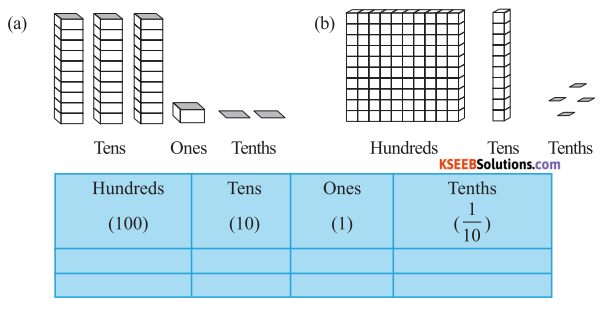Solution:
It may be observed that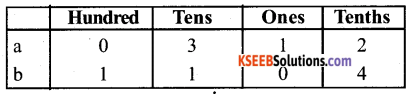To write fraction 1/2as a decimal simply divide the numerator 1 with the denominator 2 same as in the long division method.

Question 2.
Write the following decimals in the place value table
a) 19.4
b) 0.3
c) 10.6
d) 205.9
Solution: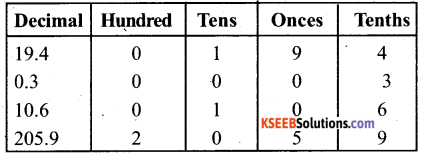What is 1/8 as a decimal? … To convert 1/8 to a decimal, divide the denominator into the numerator.

Question 3.
Write each of the following as decimals:
Solution:
a) Seven – tenthsb) Two tens and nine-tenthsc) Fourteen point six
14.6

d) One hundred and two ones
100 + 2 = 102.0

e) Six hundred point eight
600.8

Question 4.
Write each of the following as decimals:-
Solution: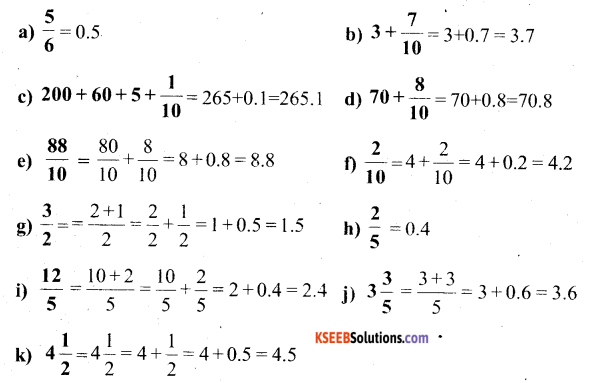Question 5.
Write the following decimals as fractions. Reduce the fractions to lowest form.
Solution: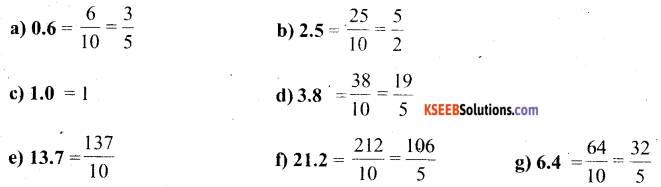Question 6.
Express the following as cm using decimals.
Solution:Question 7.
Between Which two Whole numbers on the number line are the given numbers lie? Which of these Whole numbers is nearer the number?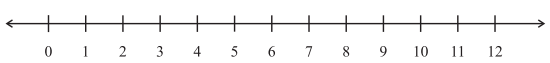a) 0.8
b) 5.1
c) 2.6
d) 6.4
e) 9.1
f) 4.9
Solution:
a) 0.8 lies between 0 and 1, and is nearest to 1.
b) 5.1 lies between 5 and 6 and is nearest to 5.
c) 2.6 lies between 2 and 3, and is near to 3.
d) 6.4 lies between 6 and 7, and is near to 6.
e) 9.1 lies between 9 and 10, and is near to 9.
f) 4.9 lies between 4 and 5, and is near to 5.

Question 8.
Show the following numbers on the number line.
a) 0.2
b) 1.9
c) 1.1
d) 2.5
Solution:
a) 0.2:- Represents a print between 0 and 1 on the numbers line. Such that the space
between 0 and 1 is divided into 10 equal parts. Hence, each equal part will be equal to one-tenth.
Now 0.2 is the second print between 0 and 1.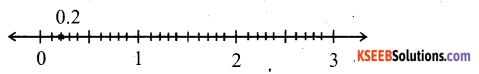b) 1.9:- Represents a print between 1 and 2 on a number line. Such that the space between 1 and 2 is divided into 10 equal parts. Hence, each part will be equal to one-tenth
Now, 1.9 is the ninth print between 1 and 2.c) 1.1 Represents a point between 1 and 2 on a number line, such that the space between 1 and 2 is divided into 10 equal parts. Hence each equal part will be equal to one-tenth Now, 1.1 is the first point between 1 and 2.d) 2.5 Represents a point between 2 and 3 on a number line, such that the space between 2
and 3 is divided into 10 equal parts. Hence each equal part will be equal to one-tenth Now, 2.5 is the fifth print between 2 and 3.Question 9.
Write the decimal number represented by the points A,B,C,D on the given number line.
Solution: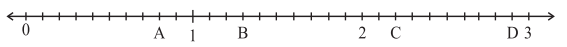Prints A, B, C, D represents 0.8, 1.3, 2.2, 2.9, respectively

Question 10.
a) The length of Ramesh’s notebook is 9 cm 5 mm What will be its length in cm?
b) The length of a young gram plant is 65 mm Express its length is in cm.
Solution:
a) The length of Ramesh’s notebook is 9 cm 5 mmb) The length of a gram plant is 65 mm